# Linear algebra, numerical methods in

The branch of numerical mathematics concerned with the mathematical description and investigation of processes for solving numerical problems in linear algebra.

Among the problems in linear algebra there are two that are the most important: the solution of a system of linear algebraic equations and the determination of the eigen values and eigen vectors of a matrix. Other problems frequently encountered are: the inversion of a matrix, the calculation of a determinant and the determination of the roots of an algebraic polynomial. These do not have, as a rule, independent significance in linear algebra and have an auxiliary character in the solution of its main problems.

Any numerical method in linear algebra can be regarded as a sequence of arithmetic operations carried out on elements of the input data. If for any input data a numerical method makes it possible to find a solution of the problem in finitely many arithmetic operations, such a method is called direct. Otherwise the numerical method is called iterative.

## Direct methods for solving a system of linear algebraic equations.

Suppose one is given a system(1)

with matrixand right-hand side. Ifis non-singular, then the solution is given by the formula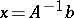, where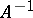is the inverse matrix to. The main idea of direct methods consists in transforming (1) to an equivalent system for which the matrix of the system is easily inverted, and consequently it is easy to find a solution of the original system. Suppose that both sides of (1) are multiplied on the left by non-singular matrices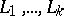. Then the new system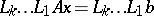(2)

is equivalent to (1). The matricescan always be chosen in such a way that the matrix on the left-hand side of (2) is sufficiently simple, for example, triangular, diagonal or unitary. In this case it is easy to calculateand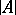.

One of the first direct methods was the Gauss method. It uses lower triangular matrices (cf. Triangular matrix)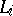and makes it possible to reduce the original system of equations to a system with an upper triangular matrix. This method is easily implemented on a computer; its scheme with choice of a principal element makes it possible to solve a system with an arbitrary matrix, and a compact scheme makes it possible to obtain results with increased accuracy by accumulating scalar products. Among the direct methods used in practice, the Gauss method requires the least amount of computational work.

Directly related to the Gauss method is Jordan's method and a modification of it, the method of optimal elimination (see ). These methods use lower and upper triangular matricesand make it possible to reduce the original system to one with a diagonal matrix. In their characteristics both methods differ little from the Gauss method, but the second makes it possible to solve a system of double the order with the same computer memory.

The stated methods belong to the group of so-called elimination methods. This name is explained by the fact that for every multiplication by a matrixone or more elements are eliminated in the matrix of the system. Elimination can be carried out not only by means of triangular matrices but also by means of unitary matrices. Various modifications of elimination methods are essentially connected with the decomposition of the matrix of the system (1) into the product of two triangular matrices or the product of a triangular and a unitary matrix.

Some methods, such as the bordering method and the completion method, are not elimination methods, although they are close to the Gauss method.

Methods based on the construction of an auxiliary system of vectors, in some way connected with the matrix of the original system and orthogonal in some metric, have been widely propagated. One of the first methods of this group was the method of orthogonal rows. The matricesin it are lower triangular and the matrix of the system (2) is unitary. Methods based on orthogonalization have many merits, but are sensitive to the influence of rounding errors. On the basis of the development of methods of orthogonalization type the very effective method (for the solution of certain systems with sparse matrices) of conjugate directions has been created (see , ).

## Direct methods for determining the eigen values and eigen vectors of a matrix.

Suppose that for a matrixit is required to determine an eigen valueand eigen vector, that is, to solve the equation(3)

Under a change of variable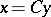, whereis a non-singular matrix, equation (3) goes over into an equation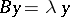with. Direct methods for solving the given problem consist of reducing the original matrixby finitely many similarity transformations to a matrixof such a simple form that it is easy to find the coefficients of the characteristic polynomial and the eigen vectors for it. The eigen values are found from the characteristic equation by one of the well-known methods. Numerical methods for the transition fromtoare essentially little different from the numerical method for transforming (1) into (2). Many methods of similarity type are known (the methods of Krylov, Danilevskii, Hessenberg, etc., see ). However, they have not been widely used in practice in view of their considerable numerical instability (see ).

One distinguishes between the complete eigen value problem, when one looks for all eigen values, and the partial problem, when one looks for some of them; the latter problem is more typical in the case of linear algebra problems which arise in the approximation of differential and integral equations via difference methods.

## Iterative methods for solving a system of linear algebraic equations.

These methods give the solution of the system (1) in the form of the limit of a sequence of certain vectors; the construction of these is carried out by a uniform process, called an iterative process. The main iterative process for the solution of (1) can be described by means of the following general scheme. Construct a sequence of vectorsfrom the recurrence formulas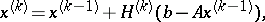where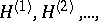is a sequence of matrices andis the initial approximation, generally speaking arbitrary. Different choices of the sequence of matrices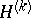lead to different iterative processes.

The simplest iterative processes are stationary processes, in which the matricesdo not depend on the step number; they are also called methods of simple iteration (see ). If the sequenceis periodic, the process is called cyclic. Every cyclic process can be transformed into a stationary process, but usually such a transformation complicates the method. Non-stationary processes, in particular cyclic processes, are used to speed up the convergence of iterative processes (see , ). Among the methods for speeding up convergence, those that use the Chebyshev polynomials (see , ) and conjugate directions (see ) take a special place.

The choice of a matrixfor a stationary process and matricesfor a non-stationary process can be made in various ways. It is possible to construct the matricesin such a way that the iterative process converges to the solution as fast as possible for a wide class of systems of equations (see ). The opposite approach is also possible, when in the construction of the matricesmaximal use is made of the peculiarities of the given system to obtain the iterative process with greatest rate of convergence (see ). The second method of constructing the matricesis more prevalent.

One of the most prevalent principles for constructing iterative processes is the relaxation principle (see Relaxation method). In this case the matricesare chosen from a class of matrices described earlier so that at each step of the process some quantity that characterizes the accuracy of the solution of the system is decreased. Among relaxation methods the ones most developed are coordinate and gradient methods. In coordinate methods the matricesare chosen so that at each step one or more components of the successive approximations change(s). For the accuracy of the approximate solutionone most frequently uses the value of the error vector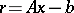.

In the case of stationary iterative methods the main error term can also be estimated by means of the-process (see ).

Among other iterative methods one can mention the simple-iteration method, the variable-directions method, the method of complete and incomplete relaxation, etc. (see , , ). As a rule, iterative methods are convenient for realization on a computer, but in contrast to direct methods they most frequently have a very restricted range of application. In their range of application iterative methods infrequently exceed direct methods substantially in the amount of computation. Therefore, the question of comparing direct and indirect methods can be solved only by a detailed study of the properties of an actual system. The most prevalent are iterative methods for solving systems that arise in the difference approximation of differential equations (see , ).

## Iterative methods for solving the complete eigen value problem.

In iterative methods the eigen values are calculated as the limits of certain numerical sequences without previously determining the coefficients of the characteristic polynomial. As a rule, one simultaneously finds the eigen vectors or certain other vectors connected with simple eigen relations. The majority of iterative methods are less sensitive to rounding errors than direct methods, but significantly more laborious. The development of these methods and the introduction of them in practical calculations became possible only after the introduction of computers.

Among iterative methods a special place is taken by the method of rotations (the Jacobi method) for solving the complete eigen value problem for a real symmetric matrix. It is based on the construction of a sequence of matrices orthogonally similar to the original matrixand such that the sum of the squares of all elements not on the main diagonal decreases monotonically to zero.

The Jacobi method is very simple, it is easily implemented on a computer and it always converges. Independently of the location of the eigen values it has asymptotic quadratic convergence. The presence of multiple and close eigen values not only does not slow down the convergence of the method, but on the contrary speeds it up. The Jacobi method is stable with respect to the influence of rounding errors in the results of intermediate calculations. The properties of this method are the reason for its prevalence in the solution of the complete eigen value problem for matrices of general form. The Jacobi method can be used, with no essential change, for Hermitian and skew-Hermitian matrices. An insignificant change in it makes it possible to solve successfully the complete eigen value problem for the matricesandat the same time, without calculating the products of the matrices themselves. An effective extension of this method to arbitrary matrices of a simple structure is realized by the generalized Jacobi method.

Among the iterative methods for solving the complete eigen value problem a significant group is formed by power methods. The majority of their computational algorithms are arranged according to the following scheme: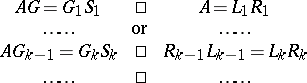Here, at each step the matrix on the left of the equality sign splits into the product of two matrices. Suppose that one of them is triangular and the other unitary or triangular of a different type. Then, under certain additional assumptions, the matricesand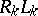converge to a quasi-triangular matrix similar to. As a rule, the orders of the diagonal cells of the quasi-triangular matrix are not large, so the complete eigen value problem for the limiting matrix is solved quite easily.

A few methods of this type are known. One of the best of them is the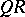-algorithm (see ).

## Investigation and classification of numerical methods.

The vast number of numerical methods of linear algebra poses an actual problem, not so much because of the creation of new methods as in the investigation and classification of existing methods (one of the most complete classifications of methods from the point of view of their mathematical structure is contained in ; the monographs , ,  are devoted to the description of methods from the point of view of their computer implementation).

The first papers on the analysis of stability and rounding errors in numerical methods for the solution of problems in linear algebra appeared only in 1947–1948 and were devoted to an investigation of the inversion of matrices and to the solution of systems of equations by methods of Gauss type. The practical value of these results was rather small. An important shift in the study of this question occurred in the middle of the 1960-s (see ), when a decisive step was taken in the analysis and estimation of equivalent perturbations. The actual computational solution of a certain problem was interpreted as the exact solution of the same problem, but corresponding to perturbations of the initial data (so-called backward analysis). This perturbation, known as equivalent perturbation, completely characterizes the influence of rounding. Many methods were investigated from the point of view of giving a majorizing estimate of the norm of the equivalent perturbation (see , ).

For a fixed computational algorithm and method of rounding the whole collection of rounding errors is a single-valued vector function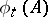, which depends on the number of digitswith which the calculation is carried out and on the original data. The principal term of the function, as, does not have any useful explicit expression. However, the investigation ofin the class of randomly specified initial data has turned out to be very effective. It was shown that the most probable value of the deviation of the computed solution from the exact solution due to rounding errors is substantially less than the maximum possible value (see ).

An analysis of the influence of rounding errors showed that there is not much fundamental difference between the best methods from the point of view of stability, i.e. with respect to rounding errors. This conclusion forces one to look in a new way at the problem of choosing some computational method in practical computational work. The creation of powerful computers substantially weakened the significance of the difference between methods in such characteristics as the size of the required computer memory and the number of arithmetical operations, and led to increased requirements to guarantee the accuracy of the solution. Under these conditions the most preferable methods were those that, while not very different from the best methods in speed and convenience of implementation on a computer, make it possible to solve a wide class of problems, both well-conditioned and ill-conditioned, and to give an estimate of the accuracy of the computed solution.

The classification and comparison of the numerical methods mentioned above refer mainly to the case when the whole collection of initial data is completely stored in the operational memory of the computer. However, there is special interest in two extreme cases — the solution of problems of linear algebra on small and on very powerful computers. The solution of problems on such computers has its own specific features.